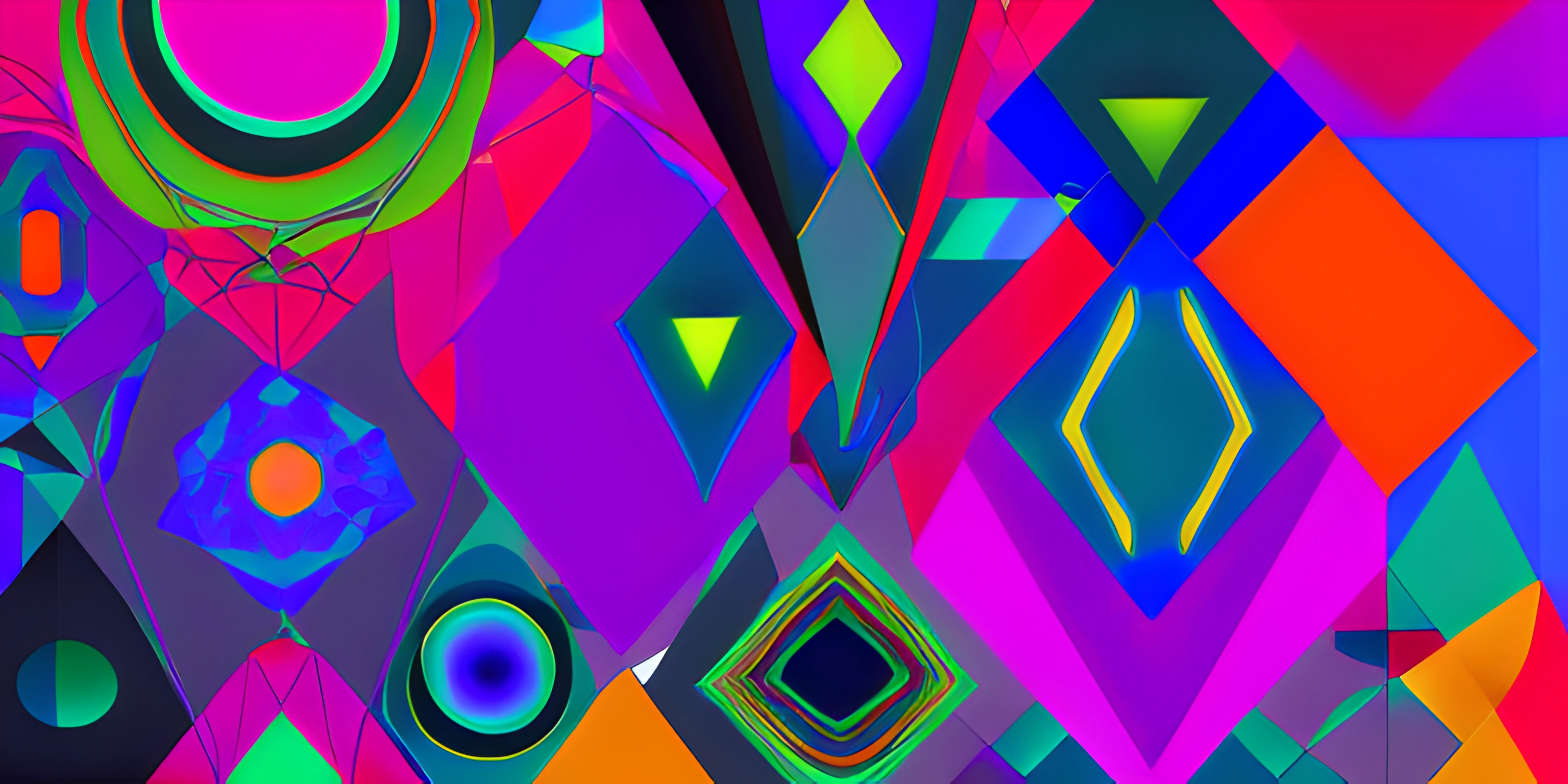# Creating Custom Shapes with p5.jsNote: this page has been created with the use of AI. Please take caution, and note that the content of this page does not necessarily reflect the opinion of Cratecode.

When diving into the world of p5.js, one of the most exciting aspects is creating custom shapes for your projects. While p5.js provides basic shapes like rectangles and circles, sometimes you need a bit more artistic freedom. That's where `beginShape` and `vertex` come in handy!

## Creating Custom Shapes with beginShape()

The `beginShape()` function in p5.js is used to initiate the creation of a complex, custom shape. It tells p5.js that the following lines of code will define the shape's vertices. After specifying all the vertices, you'll use the `endShape()` function to complete the shape definition.

Here's a basic example of using `beginShape()` and `endShape()`:

``````function setup() {
createCanvas(400, 400);
}

function draw() {
background(255);

beginShape(); // Start the shape definition
vertex(100, 100);
vertex(200, 100);
vertex(200, 200);
vertex(100, 200);
endShape(CLOSE); // End the shape definition and close the shape
}``````

This code will create a simple square on the canvas. Notice the `CLOSE` parameter in `endShape()`. This tells p5.js to connect the last vertex to the first, closing the shape.

The `vertex()` function is used to define the points (vertices) of your custom shape. It takes two arguments, the x and y coordinates of the vertex. You can add as many vertices as you need to create the desired shape.

Let's create a more interesting shape, such as a five-pointed star:

``````function setup() {
createCanvas(400, 400);
}

function draw() {
background(255);

beginShape();
vertex(200, 50);
vertex(250, 150);
vertex(350, 150);
vertex(275, 225);
vertex(300, 325);
vertex(200, 275);
vertex(100, 325);
vertex(125, 225);
vertex(50, 150);
vertex(150, 150);
endShape(CLOSE);
}``````

This code creates a five-pointed star on the canvas. The `vertex()` function is called for each point on the star, and the shape is closed with `endShape(CLOSE)`.

With `beginShape()` and `vertex()`, you can create any custom shape you can imagine. Happy shape-making!

## FAQ

### What is the basic structure for creating custom shapes in p5.js?

In p5.js, you'll be using the `beginShape()` and `vertex()` functions to create custom shapes. The basic structure looks like this:

``````function draw() {
beginShape();
vertex(x1, y1);
vertex(x2, y2);
vertex(x3, y3);
// Add more vertices as needed
endShape(CLOSE); // Use CLOSE to close the shape
}``````

### How do I create a custom triangle in p5.js?

To create a custom triangle, you'll need to define three vertices using the `vertex()` function inside the `beginShape()` and `endShape()` functions. Here's an example:

``````function draw() {
beginShape();
vertex(30, 75);
vertex(58, 20);
vertex(86, 75);
endShape(CLOSE);
}``````

### Can I use different shapes in the same sketch in p5.js?

Absolutely! You can create multiple custom shapes in your sketch by using multiple `beginShape()` and `endShape()` blocks, each defining its own set of vertices. For example:

``````function draw() {
// Custom Shape 1
beginShape();
vertex(x1, y1);
vertex(x2, y2);
vertex(x3, y3);
endShape(CLOSE);
// Custom Shape 2
beginShape();
vertex(a1, b1);
vertex(a2, b2);
vertex(a3, b3);
endShape(CLOSE);
}``````

### How do I add color and style to custom shapes in p5.js?

You can add color and style to your custom shapes by using the `fill()`, `stroke()`, and `strokeWeight()` functions before the `beginShape()` function. For example:

``````function draw() {
fill(255, 0, 0); // Red color
stroke(0, 0, 255); // Blue stroke
strokeWeight(3); // Stroke weight of 3 pixels
beginShape();
vertex(x1, y1);
vertex(x2, y2);
vertex(x3, y3);
endShape(CLOSE);
}``````

### Can I create curved custom shapes in p5.js?

Yes, you can create curved custom shapes by using the `curveVertex()` function instead of the `vertex()` function. Keep in mind that you'll need at least four vertices to create a curved shape. Here's an example:

``````function draw() {
beginShape();
curveVertex(84, 91);
curveVertex(84, 91);
curveVertex(68, 19);
curveVertex(21, 17);
curveVertex(73, 73);
curveVertex(73, 73);
endShape(CLOSE);
}``````